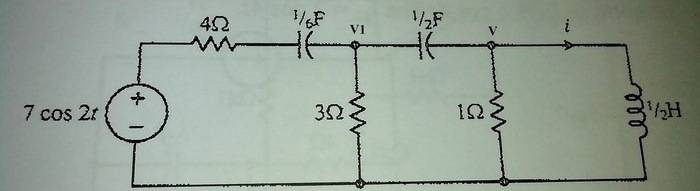# Nodal analysis question -- circuit analysis

• Engineering
• Questions999

## Homework Statement

We have the circuit in the figure.I have to find the current i using nodal analysis1/6 F =-3j
1/2 F= -j
1/2 H= j

## The Attempt at a Solution

So we have the equations
Node V1 : [(7-V1)/(4-3j) ]=[ V1/3 ]+ [(V1-V)/-j]
Node V : [(V1-V)/-j] =(V )+ (V/j)
In the V node we multiply by j each side so we have -(V1-V)=jV+V so -V1=jV
I place this in the first node so we have
In the first node we have [(7+jV)(4+3j)]/[(4-3j)(4+3j)] = -jV/3 + [(-jV-V)/-j]
We have 1.12 +0.84j-0.16jV-0.12V=-0.33JV+V-jV
Here I find V =0.1+0.85j
Now to find i I think I need to divide this by 1/2 H so by j
Problem is ,the result in my book is i=0.75 cos2t which I don't get here so where is my mistake?

## Homework Statement

We have the circuit in the figure.I have to find the current i using nodal analysis1/6 F =-3j
1/2 F= -j
1/2 H= j

## The Attempt at a Solution

So we have the equations
Node V1 : [(7-V1)/(4-3j) ]=[ V1/3 ]+ [(V1-V)/-j]
Node V : [(V1-V)/-j] =(V )+ (V/j)
In the V node we multiply by j each side so we have -(V1-V)=jV+V so -V1=jV
I place this in the first node so we have
In the first node we have [(7+jV)(4+3j)]/[(4-3j)(4+3j)] = -jV/3 + [(-jV-V)/-j]
We have 1.12 +0.84j-0.16jV-0.12V=-0.33JV+V-jV
Here I find V =0.1+0.85j
Now to find i I think I need to divide this by 1/2 H so by j
Problem is ,the result in my book is i=0.75 cos2t which I don't get here so where is my mistake?

First, the value of the left capacitor looks to be 1/6 Farad, not 1/3 Farad, right? Does that change your first equation?

Second, I prefer to write the node equations as the sum of all currents leaving the node = 0. That way I don't have to worry about signs of currents.

Could you see if you want to change the first equation, and maybe re-write the equations in the form that I suggested? That will make it a lot easier to check your work. Thanks.